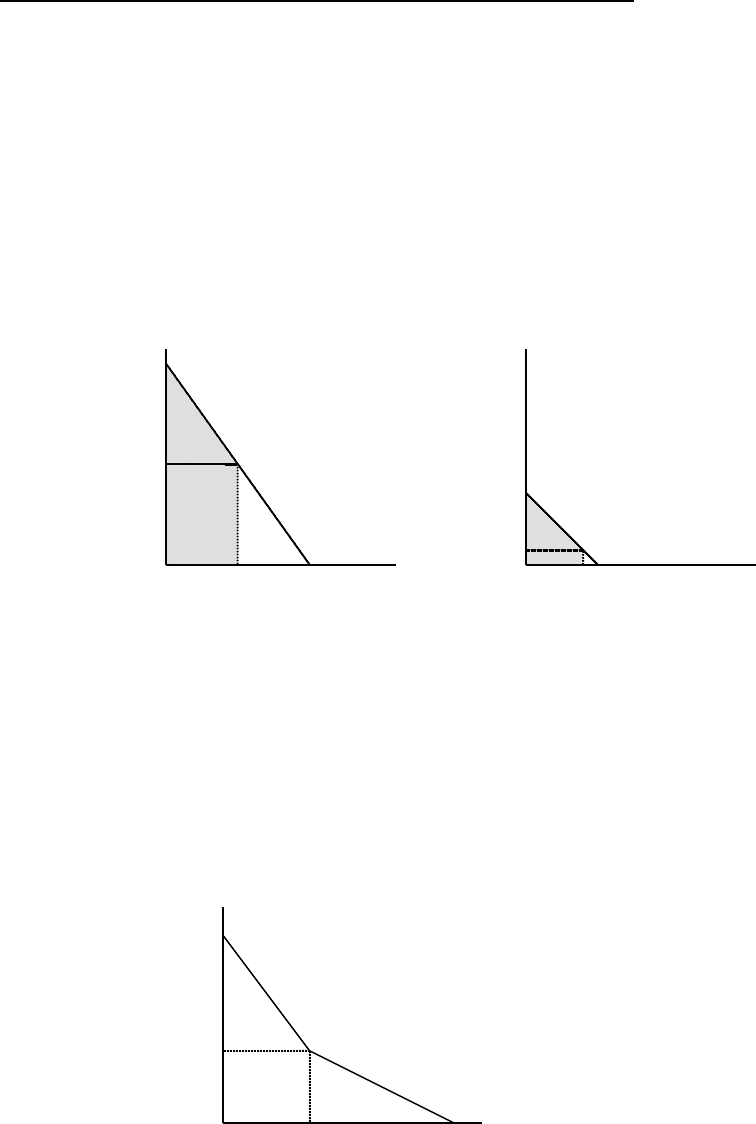# ECON 260 Chapter Notes - Chapter 3: Itap, Psoc, Pus

83 views3 pages
School
Department
Course
ProfessorChapter 3 Textbook Analytical Problem Answers
1. The individual demand curves represent the maximum amount of water that each
individual is willing to purchase at a given price. Rearrangement to isolate price
gives the maximum price each individual is willing to pay (marginal willingness to
pay; MWTP) for the Qth unit. MWTP
A
= 16-2Q
d
A
and MWTP
B
= 6-Q
d
B
.
For a
quantity of 4 units per individual, MWTP for Alvin is \$8, while MWTP for Betty is
\$2. Total WTP for Alvin is the area under his marginal WTP curve from Q
d
A
=0 to
Q
d
A
=4, and similarly for Betty. Total WTP
A
= (8
×
4)
×
[(16-8)
×
4]/2=\$48. Total
WTP
B
=(4 ×4) ×[(6-4) ×4]/2 = \$64 . Students may need to be reminded that the area of
a square is base
×
height, while the area of a triangle is (base
×
height)/2.
16
mWTP
A
mWTP
B
\$/unit \$/ unit
8
6
2
0 4 8 Q
A
0 4 6 Q
B
2. To get the aggregate demand for Alvin and Betty, add up the quantity demanded at a
given price (horizontal aggregation). The demand curve is kinked, the kink is at a
price of \$6, where Alvin purchases 5 and Betty purchases 0. The price intercept is
\$16 and the quantity intercept is 14. For a price of 0 to 6, both consumers will
demand water, aggregating the quantities demanded gives the aggregate demand
curve of Q
d
A&B
= 14-3P/2. For a price greater than \$6 only Alvin demands water,
giving an aggregate demand curve of Q
d
A&B
= 16-2P.
16
margWTP
A&B
(\$/ unit)
6
0 5 14 Q
d
A&B
Unlock document

This preview shows page 1 of the document.
Unlock all 3 pages and 3 million more documents.

## Document Summary

Chapter 3 textbook analytical problem answers: the individual demand curves represent the maximum amount of water that each individual is willing to purchase at a given price. Rearrangement to isolate price gives the maximum price each individual is willing to pay (marginal willingness to pay; mwtp) for the qth unit. For a quantity of 4 units per individual, mwtp for alvin is , while mwtp for betty is. Total wtp for alvin is the area under his marginal wtp curve from qda =0 to. Students may need to be reminded that the area of a square is base height, while the area of a triangle is (base height)/2. mwtpa. Qa: to get the aggregate demand for alvin and betty, add up the quantity demanded at a given price (horizontal aggregation). The demand curve is kinked, the kink is at a price of , where alvin purchases 5 and betty purchases 0.

# Get access

\$10 USD/m
Billed \$120 USD annually
Homework Help
Class Notes
Textbook Notes